# Partitionable graphs and odd holes

Let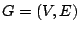be a graph, and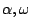arbitrary natural numbers. Assume that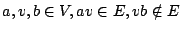are such that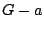,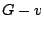have a partition of size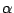into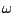-cliques, and,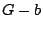have a partition of sizeinto-stable sets. It is easy to show then that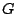is not perfect.

Given the four partitions, find an odd hole or an odd antihole.

This would imply SPGC.

This contains the following:

Given a partitionable graph (with all the partitions), find an odd hole or an odd antihole. Does the fact that the partitions are given make the task easier ?

Contributed by András Sebo

Back to the main index for Perfect Graphs.